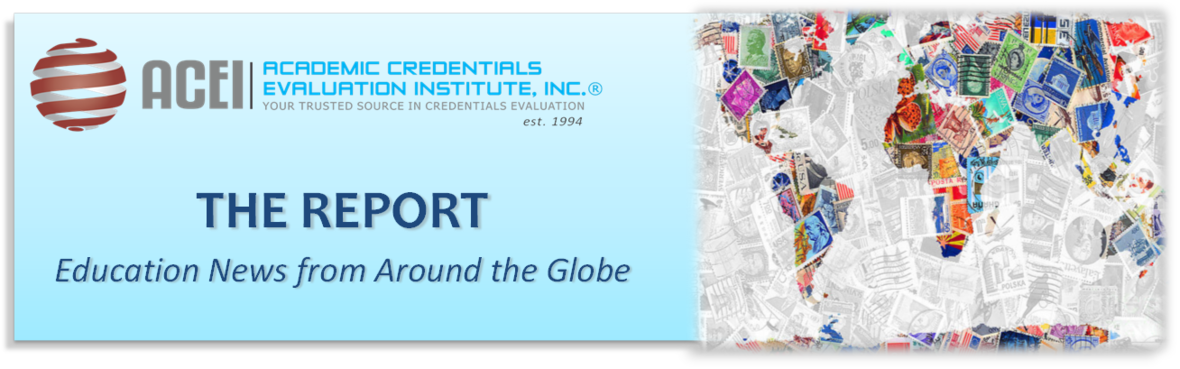# September 2021 - Los Angeles, CA

ACADEMIC CREDENTIALS EVALUATION INSTITUTE, INC. (ACEI)
Founded in 1994, ACEI is a full-service company providing complete and integrated services in-house in the areas of international education research, credential evaluation, and translation. And, through our Global Consulting Group®, we offer our expertise in the following specialties: Media and Branding, Global Pathways, and Diversity, Equity and Inclusion (DEI) to interested institutions and organizations around the globe.

 table div table+table+table div table{width:100%;padding:0}table div table+table+table div table img{width:96.23%;padding:0;float:none}table div table+table+table div table td{width:100%;padding:0 1.88% 18px}/* styles */# The Report Partners

 table div table+table+table+table+table div table{width:100%;padding:0}table div table+table+table+table+table div table table{padding:0;float:left!important;width:28.679%!important}table div table+table+table+table+table div table table+table+table td,table div table+table+table+table+table div table td{padding-left:0;padding-right:0}table div table+table+table+table+table div table table td,table div table+table+table+table+table div table table+table td{padding-left:0;padding-right:20px}table div table+table+table+table+table div table table+table{float:left!important;width:41.698%!important}table div table+table+table+table+table div table table+table+table{float:left!important;width:29.623000000000005%!important}/* styles */
 table div table+table+table+table+table+table div table{width:100%;padding:0}table div table+table+table+table+table+table div table img{width:96.23%;padding:0;float:none}table div table+table+table+table+table+table div table td{width:100%;padding:0 1.88% 18px}/* styles */# UPCOMING WEBINARS

 /* styles */ October 13, 2021 Offshore Caribbean Medical Schools Report Register here October 27,2021 ACEI GCG - STRATEGY BREAK: Getting the Change You Want at Your College/University Register here
 table div table+table+table+table+table+table+table+table+table div table{width:100%;padding:0}table div table+table+table+table+table+table+table+table+table div table img{width:96.23%;padding:0;float:none}table div table+table+table+table+table+table+table+table+table div table td{width:100%;padding:0 1.88% 18px}/* styles */# Diversity, Equity and Inclusion at Your College/University

 /* styles */ We want to learn more about what institutions like yours are doing in support of Diversity, Equity and Inclusion. Please take 30 seconds to complete our survey by October 15th and you’ll be included in our drawing for a \$50.00 Amazon gift card!
 table div table+table+table+table+table+table+table+table+table+table+table+table+table div table{width:100%;padding:0}table div table+table+table+table+table+table+table+table+table+table+table+table+table div table img{width:96.23%;padding:0;float:none}table div table+table+table+table+table+table+table+table+table+table+table+table+table div table td{width:100%;padding:0 1.88% 18px}/* styles */# Education News From Around the Globe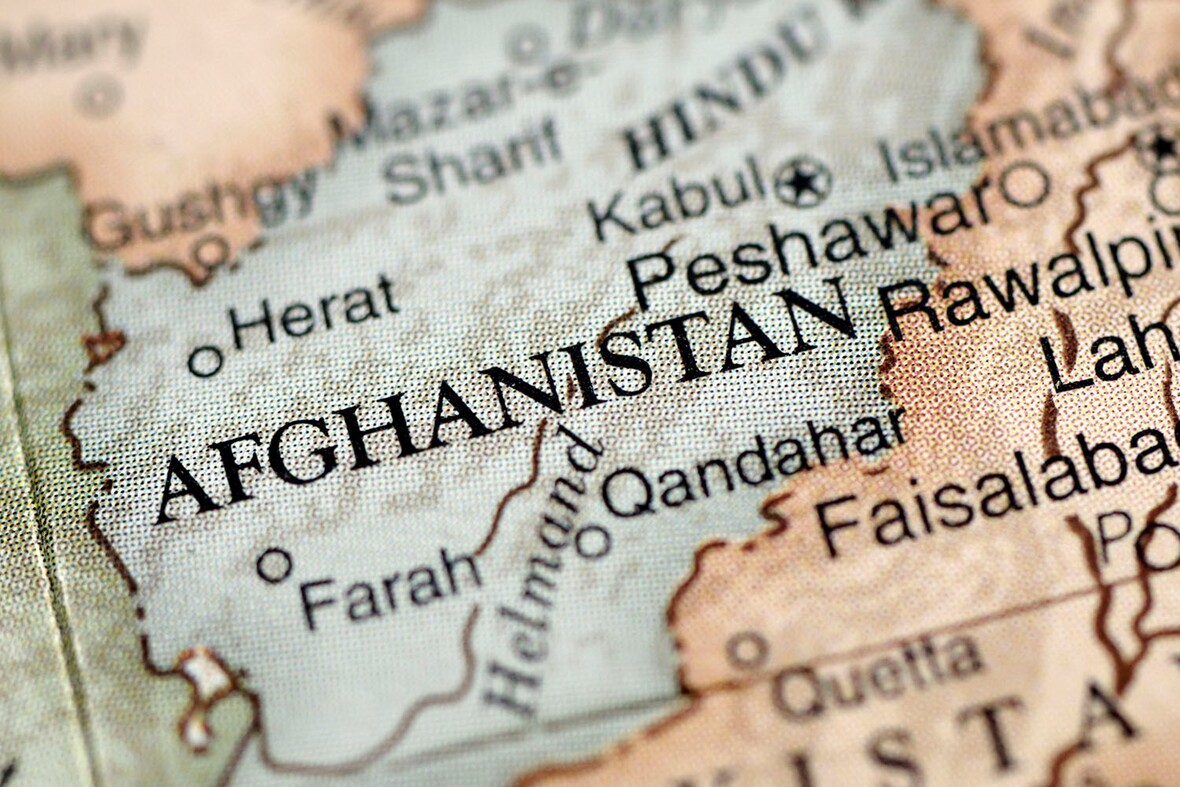# Public universities closed struggling to segregate genders

More than a month since capturing power in the Afghan capital Kabul, cementing their hold over the country, the Taliban is struggling to re-open public sector universities under a hardline gender segregation policy. To read more, click here.

 table div table+table+table+table+table+table+table+table+table+table+table+table+table+table+table+table+table div table{width:100%;padding:0}table div table+table+table+table+table+table+table+table+table+table+table+table+table+table+table+table+table div table img{width:96.23%;padding:0;float:none}table div table+table+table+table+table+table+table+table+table+table+table+table+table+table+table+table+table div table td{width:100%;padding:0 1.88% 18px}/* styles */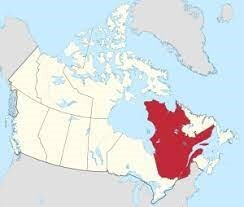# Quebec universities see cases of plagiarism double

 table div table+table+table+table+table+table+table+table+table+table+table+table+table+table+table+table+table+table+table div table{width:100%;padding:0}table div table+table+table+table+table+table+table+table+table+table+table+table+table+table+table+table+table+table+table div table img{width:96.23%;padding:0;float:none}table div table+table+table+table+table+table+table+table+table+table+table+table+table+table+table+table+table+table+table div table td{width:100%;padding:0 1.88% 18px}/* styles */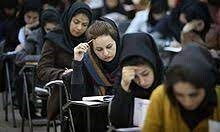# Iran rebukes UNESCO call for gender equality in education

Approx. 60% of university students in Iran are women. The hardliners in Iran are concerned about the social side-effects of the rising educational standards among women, including declining birth and marriage rates. Here's hoping that with or without the UNESCO's Education Document that calls for gender equality and inclusion in education, women will continue to have access to higher education in Iran. To read more, click here.

 table div table+table+table+table+table+table+table+table+table+table+table+table+table+table+table+table+table+table+table+table+table div table{width:100%;padding:0}table div table+table+table+table+table+table+table+table+table+table+table+table+table+table+table+table+table+table+table+table+table div table img{width:96.23%;padding:0;float:none}table div table+table+table+table+table+table+table+table+table+table+table+table+table+table+table+table+table+table+table+table+table div table td{width:100%;padding:0 1.88% 18px}/* styles */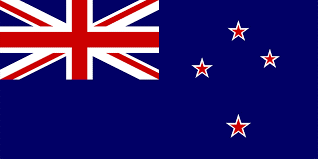# Five of eight universities see rise in cheating in 2020

According to RNZ, figures released to Checkpoint under the Official Information Act show five out of eight universities had an increase in cheating last year. Lecturers say the issue is far worse than figures show, as lockdowns disrupt valuable face to face teaching. To read more, click here.

 table div table+table+table+table+table+table+table+table+table+table+table+table+table+table+table+table+table+table+table+table+table+table+table div table{width:100%;padding:0}table div table+table+table+table+table+table+table+table+table+table+table+table+table+table+table+table+table+table+table+table+table+table+table div table img{width:96.23%;padding:0;float:none}table div table+table+table+table+table+table+table+table+table+table+table+table+table+table+table+table+table+table+table+table+table+table+table div table td{width:100%;padding:0 1.88% 18px}/* styles */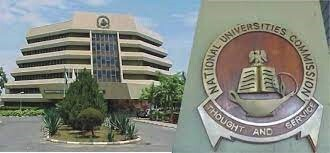# 20 new universities approved by National Universities Commission

The Federal Executive Council has, last week, approved the establishment of 20 new private universities in Nigeria, bringing their total to 99 and the total number of universities in Nigeria to 193. To read more, click here.

 table div table+table+table+table+table+table+table+table+table+table+table+table+table+table+table+table+table+table+table+table+table+table+table+table+table+table div table{width:100%;padding:0}table div table+table+table+table+table+table+table+table+table+table+table+table+table+table+table+table+table+table+table+table+table+table+table+table+table+table div table img{width:96.23%;padding:0;float:none}table div table+table+table+table+table+table+table+table+table+table+table+table+table+table+table+table+table+table+table+table+table+table+table+table+table+table div table td{width:100%;padding:0 1.88% 18px}/* styles */# How much universities charge foreigners compared to locals

Foreign students pay a significantly higher tuition in Swiss universities than locals, a new study reveals. To read more, click here.

 table div table+table+table+table+table+table+table+table+table+table+table+table+table+table+table+table+table+table+table+table+table+table+table+table+table+table+table+table div table{width:100%;padding:0}table div table+table+table+table+table+table+table+table+table+table+table+table+table+table+table+table+table+table+table+table+table+table+table+table+table+table+table+table div table img{width:96.23%;padding:0;float:none}table div table+table+table+table+table+table+table+table+table+table+table+table+table+table+table+table+table+table+table+table+table+table+table+table+table+table+table+table div table td{width:100%;padding:0 1.88% 18px}/* styles */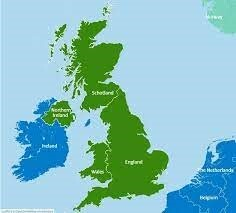# OECD warns UK universities over high fees for ‘online’ learning

British universities have been warned by the Organization for Economic Co-operation and Development (OECD) that they face a “real challenge” in justifying charging some of the highest tuition fees in the world if much of the teaching remains online. To read more, click here.

 table div table+table+table+table+table+table+table+table+table+table+table+table+table+table+table+table+table+table+table+table+table+table+table+table+table+table+table+table+table+table div table{width:100%;padding:0}table div table+table+table+table+table+table+table+table+table+table+table+table+table+table+table+table+table+table+table+table+table+table+table+table+table+table+table+table+table+table div table img{width:96.23%;padding:0;float:none}table div table+table+table+table+table+table+table+table+table+table+table+table+table+table+table+table+table+table+table+table+table+table+table+table+table+table+table+table+table+table div table td{width:100%;padding:0 1.88% 18px}/* styles */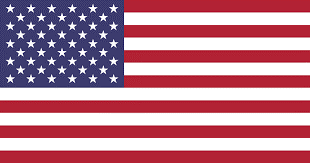# Colleges & Universities have a guy problem

American colleges and universities now enroll roughly six women for every four men. This is the largest female-male gender gap in the history of higher education, and it is getting wide. To read more, click here.

 table div table+table+table+table+table+table+table+table+table+table+table+table+table+table+table+table+table+table+table+table+table+table+table+table+table+table+table+table+table+table+table+table div table{width:100%;padding:0}table div table+table+table+table+table+table+table+table+table+table+table+table+table+table+table+table+table+table+table+table+table+table+table+table+table+table+table+table+table+table+table+table div table img{width:96.23%;padding:0;float:none}table div table+table+table+table+table+table+table+table+table+table+table+table+table+table+table+table+table+table+table+table+table+table+table+table+table+table+table+table+table+table+table+table div table td{width:100%;padding:0 1.88% 18px}/* styles */table.module-33{width:81.7%;padding:0}table div table+table+table+table+table+table+table+table+table+table+table+table+table+table+table+table+table+table+table+table+table+table+table+table+table+table+table+table+table+table+table+table+table+table div table{width:81.7%;float:none;margin-left:auto;margin-right:auto;padding:0}table div table+table+table+table+table+table+table+table+table+table+table+table+table+table+table+table+table+table+table+table+table+table+table+table+table+table+table+table+table+table+table+table+table+table div table a{border:0 none;text-decoration:none}table div table+table+table+table+table+table+table+table+table+table+table+table+table+table+table+table+table+table+table+table+table+table+table+table+table+table+table+table+table+table+table+table+table+table div table img{width:100%!important;border:0 none;text-decoration:none}table div table+table+table+table+table+table+table+table+table+table+table+table+table+table+table+table+table+table+table+table+table+table+table+table+table+table+table+table+table+table+table+table+table+table div table td{width:100%;padding:0}/* styles */

# ACEI Global Consulting Group

The Global Consulting Group is a division of ACEI that provides international education expertise with focus on Diversity, Equity, Inclusion Consulting, Global Pathway Consulting (Program/Curriculum Review for Partnerships between overseas institutions), and Media and Brand Consulting. Click here to view video presentation.

 table div table+table+table+table+table+table+table+table+table+table+table+table+table+table+table+table+table+table+table+table+table+table+table+table+table+table+table+table+table+table+table+table+table+table+table+table div table{width:100%;padding:0}table div table+table+table+table+table+table+table+table+table+table+table+table+table+table+table+table+table+table+table+table+table+table+table+table+table+table+table+table+table+table+table+table+table+table+table+table div table img{width:96.23%;padding:0;float:none}table div table+table+table+table+table+table+table+table+table+table+table+table+table+table+table+table+table+table+table+table+table+table+table+table+table+table+table+table+table+table+table+table+table+table+table+table div table td{width:100%;padding:0 1.88% 18px}/* styles *//* styles */ ACEI offers a comprehensive range of professional services that include: international credential evaluations; translations; consulting; and training. For more information on ACEI, please visit www.acei-global.org or contact: Tel: 1-310-275-3530; Email: acei@acei-global.org. ACEI was founded and incorporated in 1994 in the State of California and is a Charter and Endorsed Member of the Association of International Credential Evaluators (AICE).
 table div table+table+table+table+table+table+table+table+table+table+table+table+table+table+table+table+table+table+table+table+table+table+table+table+table+table+table+table+table+table+table+table+table+table+table+table+table+table div table{width:100%;padding:0}table div table+table+table+table+table+table+table+table+table+table+table+table+table+table+table+table+table+table+table+table+table+table+table+table+table+table+table+table+table+table+table+table+table+table+table+table+table+table div table img{width:96.23%;padding:0;float:none}table div table+table+table+table+table+table+table+table+table+table+table+table+table+table+table+table+table+table+table+table+table+table+table+table+table+table+table+table+table+table+table+table+table+table+table+table+table+table div table td{width:100%;padding:0 1.88% 18px}/* styles */# New to The Report? Click below to subscribe to this newsletter.

 Like   Tweet   Pin   +1   in